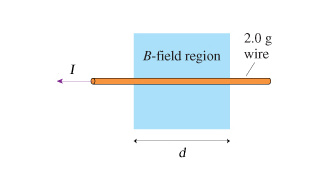# What magnetic field strength will levitate the 2.0 g wire in the figure

What magnetic field strength will levitate the 2.0 g wire in the figure(Figure 1) ? Assume that J = 1.5 A and d = 11 cm.What is the direction of the magnetic field?